Question

# 2. Hardy-Weinberg Equilibrium; chi-square test Sickle cell anemia is a recessive disorder caused by a recessive...

2. Hardy-Weinberg Equilibrium; chi-square test

Sickle cell anemia is a recessive disorder caused by a recessive mutation (S) in the b-hemoglobin gene. 80% of affected SS individuals die before reproducing.   Heterozygotes (AS) and homozygous dominant (AA) individuals do not have sickle cell anemia.

The table below shows the number of people of each genotype in a population of 100 people in population of Cameroon.

 Observed # individuals in a Cameroon population AA AS SS 62 32 6
1. What are the genotype, allele, and phenotype frequencies in this population?

b)   Is this population in Hardy-Weinberg Equilibrium? Show your work, including equations, and make sure at the end that you explain why you answered “yes” or “no” to this question.

Observed genotype frequencies:

AA = 62/100 = 0.62

AS = 32/100 = 0.32

SS = 6/100 = 0.06

Allele frequencies:

Total number of A alleles = 2*AA+AS = 2*62+32 = 156

Total number of S alleles = 2*SS +AS = 2*6+32 = 44

Total alleles = 200

Fr(A) = 156/200 = 0.78

Fr(S) = 44/200 = 0.22

Expected genotype frequencies:

AA = 0.78*0.78 = 0.6084

Number of AA individuals = 0.6084 *100 = 60.84

SS = 0.22*0.22 = 0.0484

Number of SS individuals = 0.0484 *100 = 4.84

AS = 2*0.78*0.22 = 0.3432

Number of AS individuals = 0.3432 * 100 = 34.32

 Phenotype Observed(O) Expected (E) O-E (O-E)2 (O-E)2/E AA 62 60.84 1.16 1.35 0.022 AS 32 34.32 -2.32 5.38 0.157 SS 6 4.84 1.16 1.35 0.278 100 100 0.457

Chi-square value = 0.457

Degrees of freedom = Number of phenotypes – 1

Df = 3-1=2

Chi-square value of 0.457 is lesser than the critical value of 5.99. We can accept the null hypothesis and the population is in Hardy-Weinberg equilibrium.

#### Earn Coins

Coins can be redeemed for fabulous gifts.

Similar Homework Help Questions
• ### A mutation in one of the hemoglobin genes causes sickle cell anemia. The sickle cell allele,...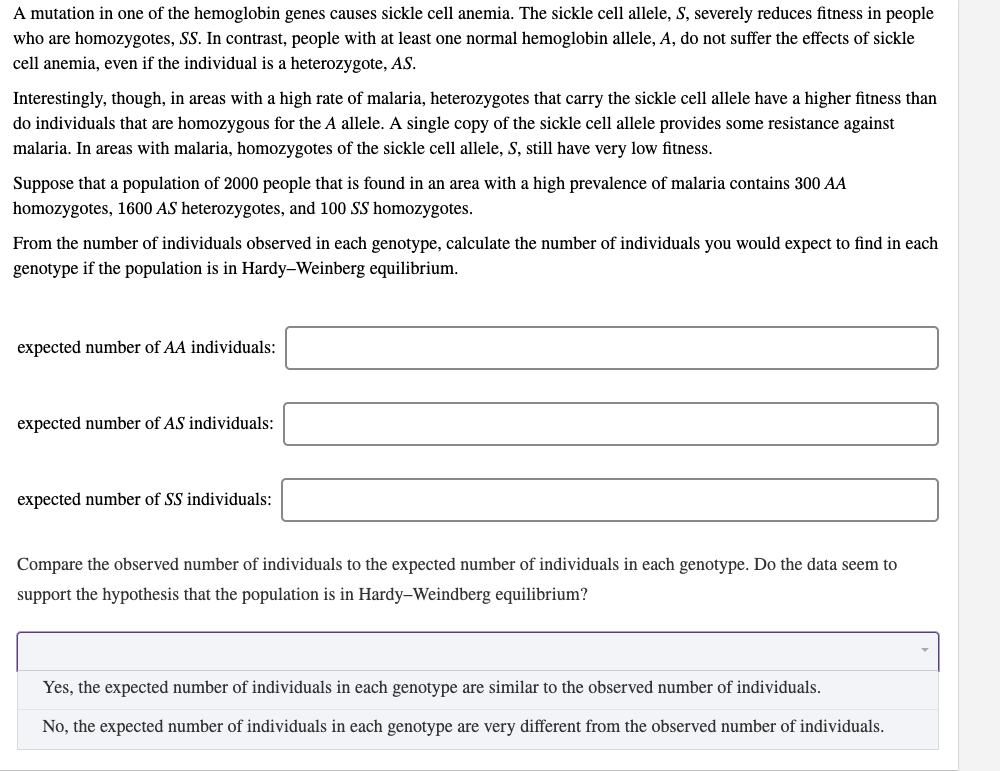A mutation in one of the hemoglobin genes causes sickle cell anemia. The sickle cell allele, S, severely reduces fitness in people who are homozygotes, SS. In contrast, people with at least one normal hemoglobin allele, A, do not suffer the effects of sickle cell anemia, even if the individual is a heterozygote, AS. Interestingly, though, in areas with a high rate of malaria, heterozygotes that carry the sickle cell allele have a higher fitness than do individuals that are...

• ### A mutation in one of the hemoglobin genes causes sickle cell anemia. The sickle cell allele,...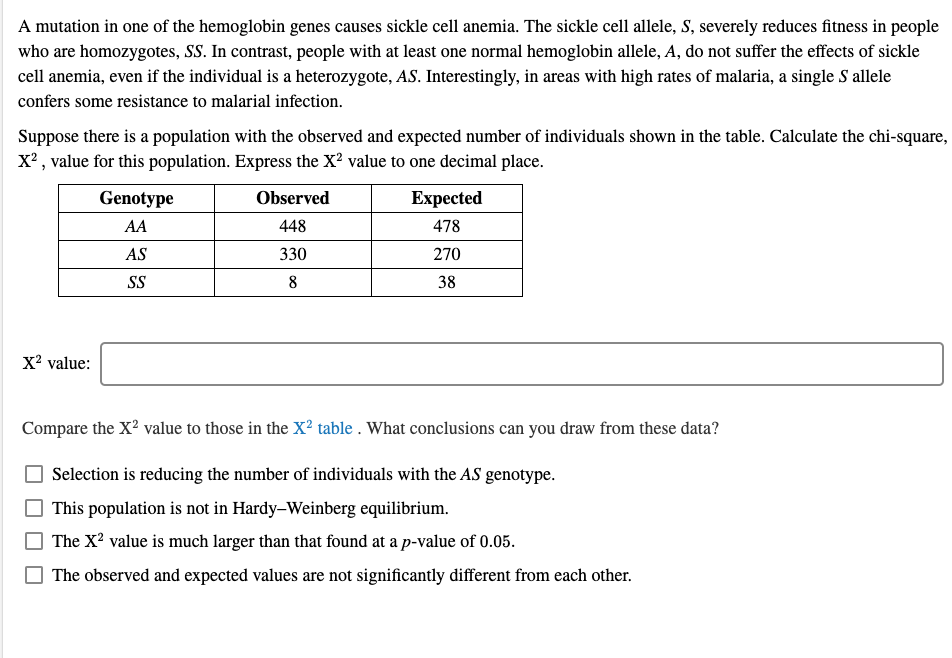A mutation in one of the hemoglobin genes causes sickle cell anemia. The sickle cell allele, S, severely reduces fitness in people who are homozygotes, SS. In contrast, people with at least one normal hemoglobin allele, A, do not suffer the effects of sickle cell anemia, even if the individual is a heterozygote, AS. Interestingly, in areas with high rates of malaria, a single Sallele confers some resistance to malarial infection. Suppose there is a population with the observed and...

• ### In humans, sickle-cell anemia (HBBSHBBS) is caused by the recessive condition of an autosomal gene with...

In humans, sickle-cell anemia (HBBSHBBS) is caused by the recessive condition of an autosomal gene with two alleles: HBBA (wild-type) and HBBS (sickle-cell mutation). In one population, the frequency of individuals who do not suffer from sickle-cell anemia (HBBAHBBA and HBBAHBBS) is 0.9964. Assuming the population is in Hardy-Weinberg equilibrium, what is the frequency of the HBBS allele?

• ### Hardy-Weinberg Practice Problems: You need to list equations used and provide steps of problem solving. Providing...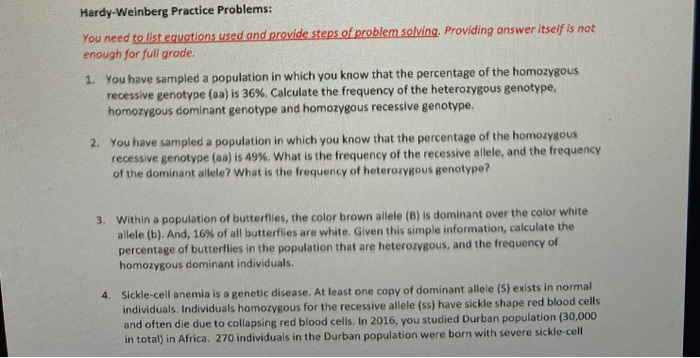Hardy-Weinberg Practice Problems: You need to list equations used and provide steps of problem solving. Providing answer itself is not enough for full grade. 1. You have sampled a population in which you know that the percentage of the homozygous recessive genotype (aa) is 36%. Calculate the frequency of the heterozygous genotype, homozygous dominant genotype and homozygous recessive genotype. 2. You have sampled a population in which you know that the percentage of the homozygous recessive genotype (aa) is 49%....

• ### In humans, sickle-cell anemia is caused by a recessive lethal allele Hbs. A healthy allele is...

In humans, sickle-cell anemia is caused by a recessive lethal allele Hbs. A healthy allele is denoted by Hba. Following is a table that shows the three possible genotypes, with the associated phenotypes. Genotype Phenotype Hba Hba Healthy (no sign of disease) HbaHbs Healthy (no sign of disease) Hbs Hbs Shows symptoms of sickle-cell anemia What is the probability of two heterozygous individuals giving birth to a child who has a sickle-cell allele, but shows a healthy phenotype? Enter in...

• ### In a population that is in Hardy Weinberg equilibrium, 49% of the individuals are recessive homozygotes...

In a population that is in Hardy Weinberg equilibrium, 49% of the individuals are recessive homozygotes for a certain trait. In a population of 5500, calculate the following: a) The number of homozygous recessive individuals in the population b) The number of heterozygotes in the population

• ### please click on the photo to see all of it The basic equations of Hardy-Weinberg Equilibrium...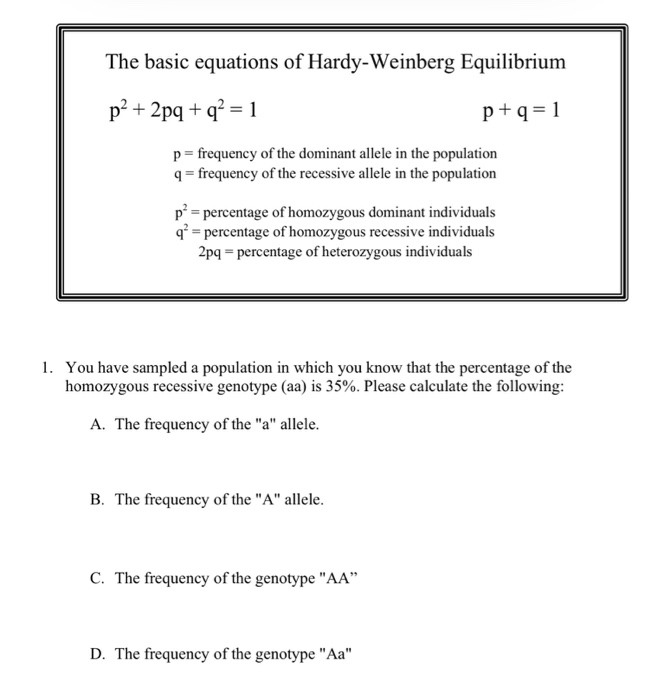please click on the photo to see all of it The basic equations of Hardy-Weinberg Equilibrium p² + 2pq + q2 = 1 p+q=1 p= frequency of the dominant allele in the population 9 = frequency of the recessive allele in the population př= percentage of homozygous dominant individuals q* = percentage of homozygous recessive individuals 2pq - percentage of heterozygous individuals 1. You have sampled a population in which you know that the percentage of the homozygous recessive genotype...

• ### 2.3 Problem 3 The Hardy-Weinberg equation is useful for predicting the percent of a hu- man...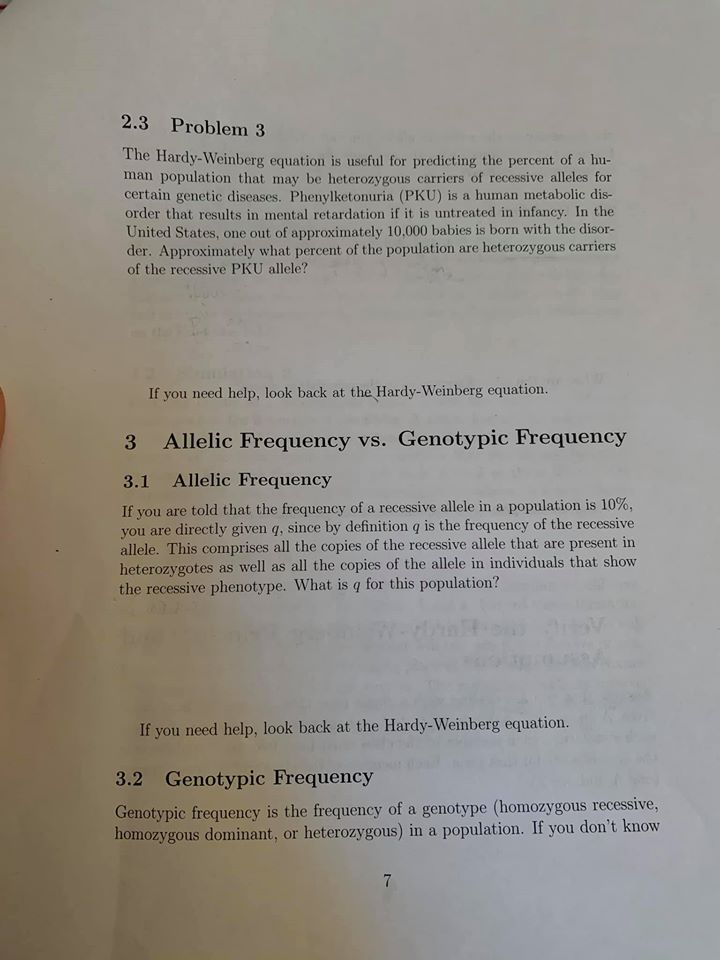2.3 Problem 3 The Hardy-Weinberg equation is useful for predicting the percent of a hu- man population that may be heterozygous carriers of recessive alleles for certain genetic diseases. Phenylketonuria (PKU) is a human metabolic dis- order that results in mental retardation if it is untreated in infancy. In the United States, one out of approximately 10.000 babies is born with the disor- der. Approximately what percent of the population are heterozygous carriers of the recessive PKU allele? If you...

• ### Evolution 1. Assume this population is in Hardy-Weinberg equilibrium. In a population of 120 cats, 35...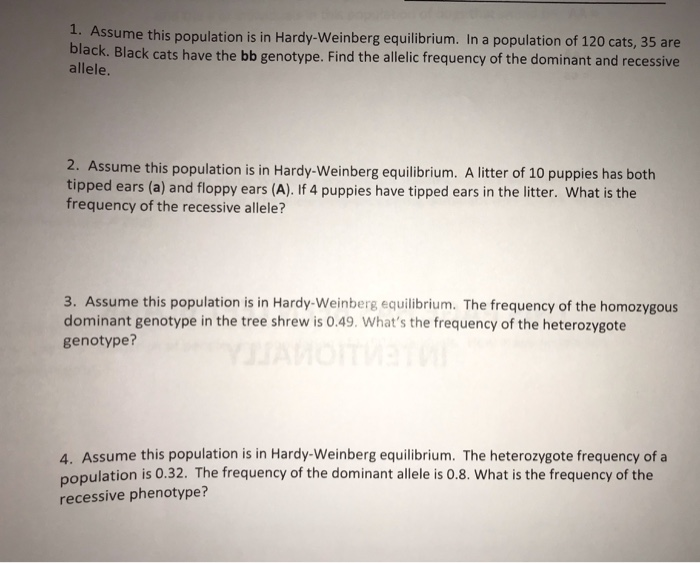Evolution 1. Assume this population is in Hardy-Weinberg equilibrium. In a population of 120 cats, 35 are black. Black cats have the bb genotype. Find the allelic frequency of the dominant and recessive allele. 2. Assume this population is in Hardy-Weinberg equilibrium. A litter of 10 puppies has both tipped ears (a) and floppy ears (A). If 4 puppies have tipped ears in the litter. What is the frequency of the recessive allele? 3. Assume this population is in Hardy-Weinberg...

• ### Sickle-cell anemia is caused by a single point mutation in the hemoglobin of human red blood cells. It is a recessive gene. People who have sickle cell anemia are homozygous recessive and suffer terribly from this disorde

Sickle-cell anemia is caused by a single point mutation in the hemoglobin of human red blood cells.  It is a recessive gene.  People who have sickle cell anemia are homozygous recessive and suffer terribly from this disorder.  Now I have stated in lecture that mutations are neither good nor bad.  Rather it depends on how the mutation effects the survival of the species.  If the mutation harms the survival of the species it is bad, if it helps the survival...